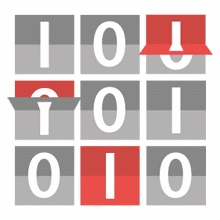# BRICS - Bit Flip Resilience for In-Memory Column Stores

Research project on detecting and correcting transient hardware errors (bit flips) during query processing in main-memory-centric database systems (especially, column stores).

## AN Coding

The core of our techniques for detecting transient hardware errors (multi-bit flips) in software are based on an arithmetic coding called AN Coding. It requires to encode each and every integer value and consequently allows to detect bit flips in each and every integer value, as well. AN Coding only works on integers, but this is not a limitation, because we can view any information as a single integer or a sequence of integers (e.g. strings).

Arithmetic codes were first mentioned in the mid-1950s when Diamond proposed one form of AN coding . Shortly after, his work was extended by Brown in 1960 . Later, AN codes were developed for detecting errors in processors, mainly in the field of embedded systems , . As we noted above, AN codes received quite some attention during the previous decade and was even proposed to be used in the automotive domain .

There are other domains which use coding operations, too, like compression and security. There, encoding is called compress and encrypt, respectively, while decoding is called decompress and decrypt. To distinguish from these domains, we use different terms: hardening for increasing the code strength and softening for reducing the code strength. We chose these terms since they underline the fact that data is literally hardened (or softened) against bit flips. We will still use “encoding” and “decoding” to add or remove code bits, respectively.

### Coding Operations

Suppose we have some arbitrary integer (data) $d$ which we want to encode. AN coding works by multiplying a constant $A$ onto the data unit $d$:

Encoding Decoding Error Detection
Original $d \cdot A = c$ $d = c / A$ $c \% A \equiv 0 \rightarrow$ valid
$c \% A \not\equiv 0 \rightarrow$ invalid

The Original coding operations are what used to be the standard way. Obviously, the division (decoding) and modulus (error detection) operations are painfully slow, even on modern CPUs. We realized that by restricting the parameter $A$ to odd values only, we can use the modular multiplicative inverse of $A$, which results in the Improved coding operations for decoding and error detection shown just below. This works, because CPUs implicitly compute in residue class rings, or quotient rings, which means that any arithmetic operation on integers is implicitly done $\text{mod} 2^n$, where $n$ is the number of data bits (i.e. register width). To the best of our knowledge, we are the first in more than 60 years since the first mentioning of AN codes to publish this nice mathematical solution.

Encoding Decoding Error Detection
Improved $d \cdot A = c$ $d = c \cdot A^{-1}$ $% $ invalid
$c \cdot A^{-1} = d^* > d_\text{max} \rightarrow$ invalid
otherwise $\rightarrow$ valid

Using the inverse works, because CPUs’ ALUs implicitly compute in residue class rings. This means that any arithemtic operation is implicitly $\text{mod } 2^n$, where $n$ is the data (register) width in bits, i.e. typically $8$, $16$, $32$, or $64$. For the improved error detection, we need to know the exact data type of $d$. From that, we know the smallest and largest encodable values from the original data domain.

1. Checking codes for digital computers

J. M. Diamond

Proceedings of the IRE, vol. 43, no. 4, pp. 487–488, Apr. 1955.

2. Error detecting and correcting binary codes for arithmetic operations

D. T. Brown

IRE Transactions on Electronic Computers, vol. EC-9, no. 3, pp. 333–337, Sep. 1960.

3. Vital coded microprocessor: Principles and application for various transit systems

P. Forin

IFAC-GCCT, pp. 79–84, Sep. 1989.

4. Software Encoded Processing: Building Dependable Systems with Commodity Hardware

Ute Wappler, Christof Fetzer

International Conference on Computer Safety, Reliability, and Security, pp. 356-369, Sep. 2007.